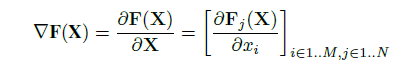# How to compute Jacobian matrix in PyTorch?

#1

For one of my tasks, I am required to compute a forward derivative of output (not loss function) w.r.t given input X. Mathematically, It would look like this:Which is essential a Jacobian of the output. It is different from backpropagation in two ways. First, we want derivative of network output not the loss function. Second, It is calculated w.r.t to input X rather than network parameters. I think this can be achieved in Tensorflow using `tf.gradients()`. How do I perform this op in PyTorch? I am not sure if I can use `backward()` function here.

Thanks

4 Likes
(jpeg729) #2

You can use torch.autograd.grad for stuff like this.

1 Like
#3

Hi, I think to this day the only way is to use grad function, but you will need to call it j times (once for each output). Unfortunately this requires many backward propagations and scales terribly with the output space of the function.

I have this exact same need, is there a different way to get the Jacobian of a function?

1 Like
#4

I came across a different solution which uses `backward` function. It’s all about playing with `backward`'s parameters. More information can be found here. I am still looking for a better solution, if it exists.

1 Like
#5

Thank you saan77, but I am still unable to understand how it is possible to get the Jacobian with a single backward pass.

The `grad_tensors` argument of backward seems to work as a weighting mask for the `tensors` argument in the thread you posted.

I don’t want the gradient of my tensors to be accumulated at leaf nodes. I want to get the gradient of each of my tensors with respect to leaf nodes. Let’s say I have an image classifier, whose input has shape (batchsize, c, h, w) and its output has shape (batchsize, n_classes), I want the jacobian to have shape (batchsize, c, h, w, n_classes).

Did you manage to get something similar?

#6

Yes, you need to call it n times, where n is the number of output nodes. I am not sure if there is a way to compute this in a single pass. `compute_jacobian` function of this script computes jacobian using `backward`.

(Saurabh) #7

I have the exact same issue. I need to compute jacobian many times, and it’s terribly slow to have that many backward passes.

The python Autograd library is much better for jacobian. I was thinking if I could do the same with pytorch.

I hope they implement jacobian soon.

2 Likes
(Chi Po Choi) #8

I guess it is related to “reverse-mode vs forward-mode”. As wikipedia Automatic_differentiation states, reverse-mode is more efficient for “tensor input scalar output” while forward-mode is more efficient for “scalar input tensor output”. That’s why machine learning library uses reverse-mode.

Jacobian matrix, however, is about “tensor input tensor output”. Not sure which way would be more efficient.

1 Like
(Shane Barratt) #9

The following code will do the trick with a single call to backward, taking advantage of when the function takes batched inputs.

1 Like
(phizaz) #10

Interesting, I think it only works with input vectors, I don’t see a way to extend it to parameter vectors.